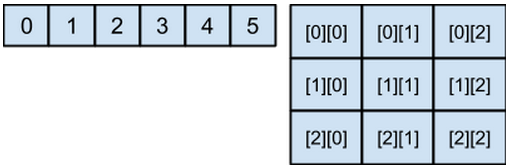# Arrays, comments and conventions for identifiers

## Arrays in Java

The array is an ordered sequence of a type of data. We can think of it, as a numbered row of variables of the same type. The type used in the array may be a primitive type, a class or another array. Using arrays of arrays, we can construct tables or matrices of two or more dimensions:The array type is defined, by specifying the type used in the array, followed by []. In the case of a two-dimensional table we use: [] [].

Examples of array declarations:

```    String[] word;
int[] numbers;
int[][] matrix;
Person people[];
```

There are also different ways to initialize an array:

```    String fruits[] = {"apple","pear","banana"}; // initialized with a constant array
int[] numbers= new int; // initialized with ten zeros
int[][] matrix = new int; // initializes a 3 by 3 table, with each of the cells with a zero
Person[] people = new Person;
```

In the case of fruits, it is initialized with a constant array. A constant array, is simply a list of values written in ​​between {}, separated by commas.

In the case of the variable numbers, it is initialized with ten boxes with an int type in each box, with its default value; zero.

It is important to take into account that Java arrays are numbered from zero. Let's see how to access every element of the array:

```    String fruit = fruits; // Assigns the fruit variable with the constant "apple"
numbers  = 3; / / Assign a three, to the fifth position in the array numbers
matrixA   = -1; / / Assigns the lower right corner of the table, a -1     people  = new Person (); / / Assign a new object person,  to the second position of the array people
```

The rest are initialized with the default values ​​for each type; int with zeros and people with null.

## Array length property

Arrays objects have a field called length, indicating how many positions they have.

```	int[] numbers= new int;
int length = numbers.length; // the value of length is 10String
fruits[] = {"apple","pear","banana"};
int numberFruits = fruits.length // the value of numberFruits is 3 ```

This is an introduction to Arrays. We will see more about how to handle Arrays and other more powerful containers, such as collections, after learning the "for" statement.

Comments are ignored by the compiler. They are used to explain the source code without affecting the functionality of the code.

In the examples above, we can see how, at the end of each line, a comment is added using / /. Everything written in a line after / / is a comment and is ignored by the compiler.

There is a second type of comments, which can include multiple lines. Everything between / * and * / is a comment and is ignored by the compiler, no matter how many lines there is in between.

## Naming conventions for Java identifiers

Identifiers are the names we choose for classes, variables, methods, etc.. In Java, there is a convention on how to name identifiers:

• If the identifier is composed of more than one word:

• for constants, the words are separated by underscores "_"